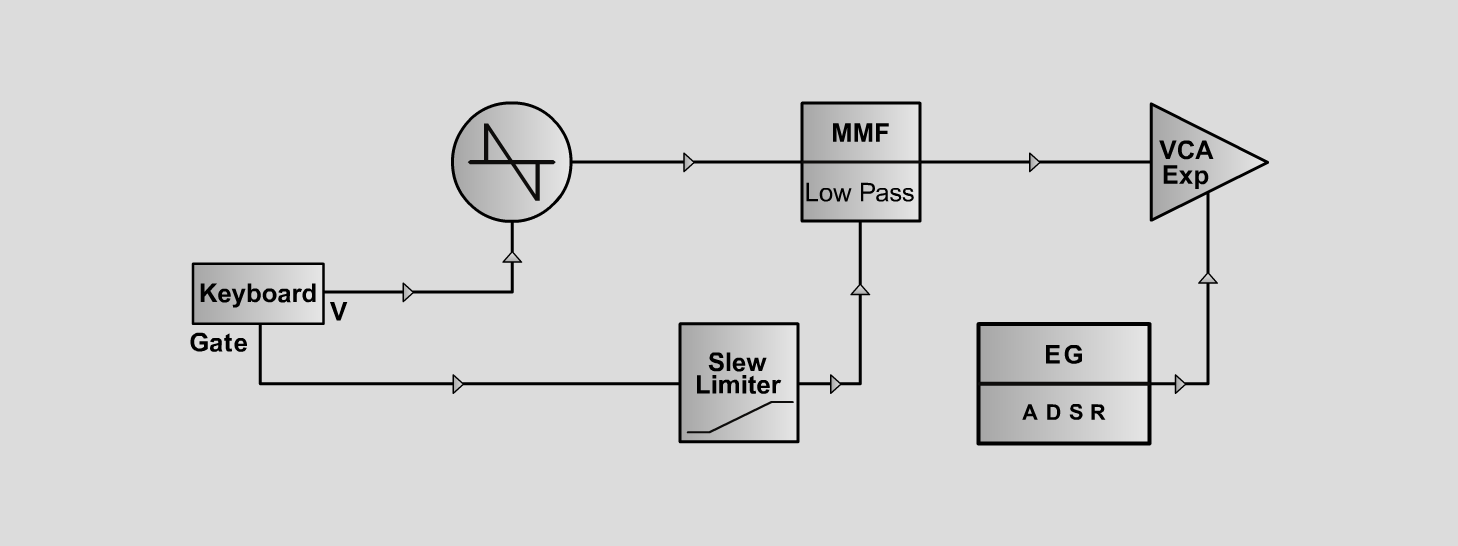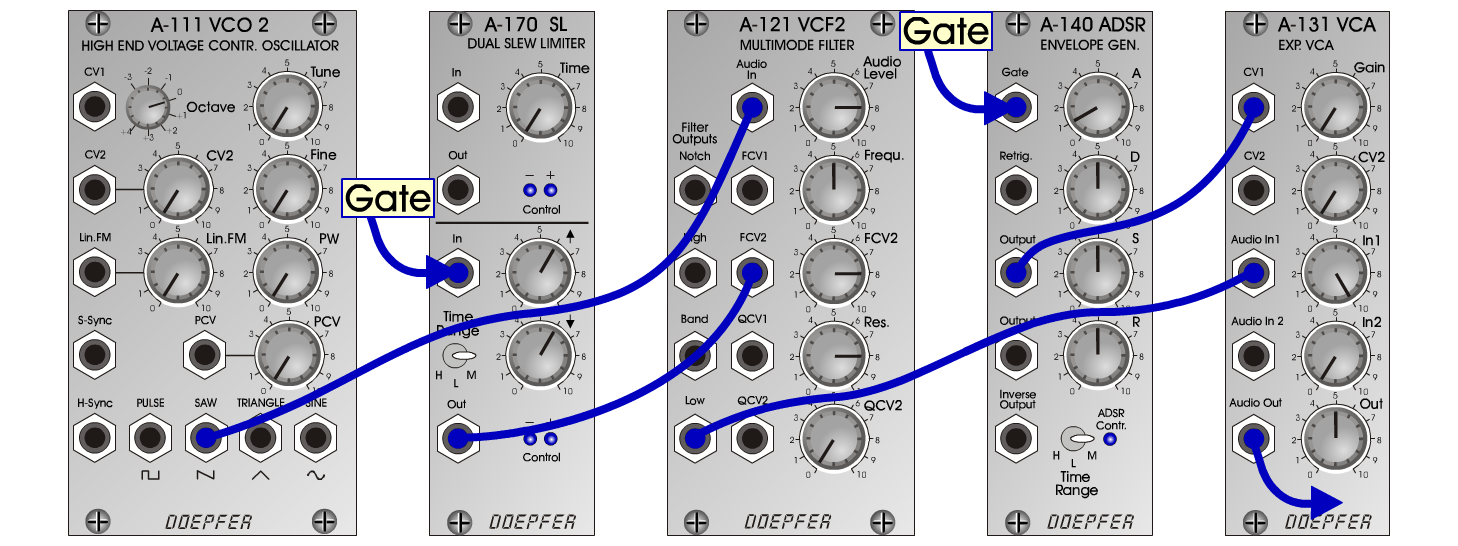Comic-VoiceVoicesC o m i c - V o i c e Connections: Settings: A-111 (Saw)  <=>  A-121 (Audio In)  A-121 (Low)  <=>  A-131 (Audio In)  A-140 (Output)  <=>  A-131 (CV 1)  KB (Gate)  <=>  A-170/Lower (In)  A-170 (Out)  <=>  A-121 (FCV 2)  A-131 (Audio Out)  <=> Amplifier A-121 (Audio Level = 10, Freq = 2, FCV 2 = 6, Res = 8)  A-140 (A = 1, D = 5, S = 5, R = 5, Range = M)  A-170 (Time/up = 6, Time/down = 6, Range = M)  A-131 (Gain = 0, Audio In 1 = 10,  Audio Out = 5)Sound samples Limoges (with X-Delay)     (Mussorgski: Pictures at an Exhibition) Comic-Voice (65 Hz) Comic-Voice (130 Hz) Comic-Voice (261 Hz) Comic-Voice (523 Hz)